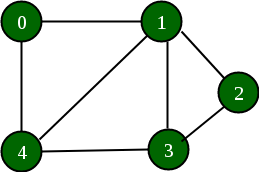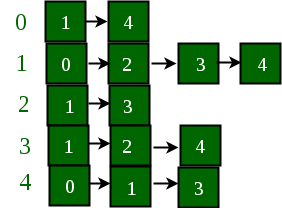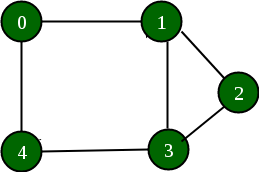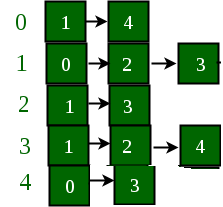# Add and Remove Edge in Adjacency List representation of a Graph

Prerequisites: Graph and Its Representation

A vector has been used to implement the graph using adjacency list representation. It is used to store the adjacency lists of all the vertices. The vertex number is used as the index in this vector.

Example:

Below is a graph and its adjacency list representation:If the edge between 1 and 4 has to be removed, then the above graph and the adjacency list transforms to:## Recommended: Please try your approach on {IDE} first, before moving on to the solution.

Approach: The idea is to represent the graph as an array of vectors such that every vector represents adjacency list of the vertex.

• Adding an edge: Adding an edge is done by inserting both of the vertices connected by that edge in each others list. For example, if an edge between (u, v) has to be added, then u is stored in v’s vector list and v is stored in u’s vector list. (push_back)
• Deleting an edge: To delete edge between (u, v), u’s adjacency list is traversed until v is found and it is removed from it. The same operation is performed for v.(erase)

Below is the implementation of the approach:

## C++

 `// C++ implementation of the above approach ` ` `  `#include ` `using` `namespace` `std; ` ` `  `// A utility function to add an edge in an ` `// undirected graph. ` `void` `addEdge(vector<``int``> adj[], ``int` `u, ``int` `v) ` `{ ` `    ``adj[u].push_back(v); ` `    ``adj[v].push_back(u); ` `} ` ` `  `// A utility function to delete an edge in an ` `// undirected graph. ` `void` `delEdge(vector<``int``> adj[], ``int` `u, ``int` `v) ` `{ ` `    ``// Traversing through the first vector list ` `    ``// and removing the second element from it ` `    ``for` `(``int` `i = 0; i < adj[u].size(); i++) { ` `        ``if` `(adj[u][i] == v) { ` `            ``adj[u].erase(adj[u].begin() + i); ` `            ``break``; ` `        ``} ` `    ``} ` ` `  `    ``// Traversing through the second vector list ` `    ``// and removing the first element from it ` `    ``for` `(``int` `i = 0; i < adj[v].size(); i++) { ` `        ``if` `(adj[v][i] == u) { ` `            ``adj[v].erase(adj[v].begin() + i); ` `            ``break``; ` `        ``} ` `    ``} ` `} ` ` `  `// A utility function to print the adjacency list ` `// representation of graph ` `void` `printGraph(vector<``int``> adj[], ``int` `V) ` `{ ` `    ``for` `(``int` `v = 0; v < V; ++v) { ` `        ``cout << ``"vertex "` `<< v << ``" "``; ` `        ``for` `(``auto` `x : adj[v]) ` `            ``cout << ``"-> "` `<< x; ` `        ``printf``(``"\n"``); ` `    ``} ` `    ``printf``(``"\n"``); ` `} ` ` `  `// Driver code ` `int` `main() ` `{ ` `    ``int` `V = 5; ` `    ``vector<``int``> adj[V]; ` ` `  `    ``// Adding edge as shown in the example figure ` `    ``addEdge(adj, 0, 1); ` `    ``addEdge(adj, 0, 4); ` `    ``addEdge(adj, 1, 2); ` `    ``addEdge(adj, 1, 3); ` `    ``addEdge(adj, 1, 4); ` `    ``addEdge(adj, 2, 3); ` `    ``addEdge(adj, 3, 4); ` ` `  `    ``// Printing adjacency matrix ` `    ``printGraph(adj, V); ` ` `  `    ``// Deleting edge (1, 4) ` `    ``// as shown in the example figure ` `    ``delEdge(adj, 1, 4); ` ` `  `    ``// Printing adjacency matrix ` `    ``printGraph(adj, V); ` ` `  `    ``return` `0; ` `} `

## Java

 `// Java implementation of the above approach ` `import` `java.util.*; ` ` `  `class` `GFG ` `{ ` ` `  `// A utility function to add an edge in an ` `// undirected graph. ` `static` `void` `addEdge(Vector adj[], ` `                    ``int` `u, ``int` `v) ` `{ ` `    ``adj[u].add(v); ` `    ``adj[v].add(u); ` `} ` ` `  `// A utility function to delete an edge in an ` `// undirected graph. ` `static` `void` `delEdge(Vector adj[], ` `                    ``int` `u, ``int` `v) ` `{ ` `    ``// Traversing through the first vector list ` `    ``// and removing the second element from it ` `    ``for` `(``int` `i = ``0``; i < adj[u].size(); i++)  ` `    ``{ ` `        ``if` `(adj[u].get(i) == v)  ` `        ``{ ` `            ``adj[u].remove(i); ` `            ``break``; ` `        ``} ` `    ``} ` ` `  `    ``// Traversing through the second vector list ` `    ``// and removing the first element from it ` `    ``for` `(``int` `i = ``0``; i < adj[v].size(); i++) ` `    ``{ ` `        ``if` `(adj[v].get(i) == u) ` `        ``{ ` `            ``adj[v].remove(i); ` `            ``break``; ` `        ``} ` `    ``} ` `} ` ` `  `// A utility function to print the adjacency list ` `// representation of graph ` `static` `void` `printGraph(Vector adj[], ``int` `V) ` `{ ` `    ``for` `(``int` `v = ``0``; v < V; ++v) ` `    ``{ ` `        ``System.out.print(``"vertex "` `+ v+ ``" "``); ` `        ``for` `(Integer x : adj[v]) ` `            ``System.out.print(``"-> "` `+ x); ` `        ``System.out.printf(``"\n"``); ` `    ``} ` `    ``System.out.printf(``"\n"``); ` `} ` ` `  `// Driver code ` `public` `static` `void` `main(String[] args) ` `{ ` `    ``int` `V = ``5``; ` `    ``Vector []adj = ``new` `Vector[V]; ` `        ``for` `(``int` `i = ``0``; i < V; i++) ` `            ``adj[i] = ``new` `Vector(); ` `     `  `    ``// Adding edge as shown in the example figure ` `    ``addEdge(adj, ``0``, ``1``); ` `    ``addEdge(adj, ``0``, ``4``); ` `    ``addEdge(adj, ``1``, ``2``); ` `    ``addEdge(adj, ``1``, ``3``); ` `    ``addEdge(adj, ``1``, ``4``); ` `    ``addEdge(adj, ``2``, ``3``); ` `    ``addEdge(adj, ``3``, ``4``); ` ` `  `    ``// Printing adjacency matrix ` `    ``printGraph(adj, V); ` ` `  `    ``// Deleting edge (1, 4) ` `    ``// as shown in the example figure ` `    ``delEdge(adj, ``1``, ``4``); ` ` `  `    ``// Printing adjacency matrix ` `    ``printGraph(adj, V); ` `} ` `} ` ` `  `// This code is contributed by 29AjayKumar `

## C#

 `// C# implementation of the above approach ` `using` `System; ` `using` `System.Collections.Generic; ` ` `  `class` `GFG ` `{ ` ` `  `// A utility function to add an edge in an ` `// undirected graph. ` `static` `void` `addEdge(List<``int``> []adj, ` `                    ``int` `u, ``int` `v) ` `{ ` `    ``adj[u].Add(v); ` `    ``adj[v].Add(u); ` `} ` ` `  `// A utility function to delete an edge in an ` `// undirected graph. ` `static` `void` `delEdge(List<``int``> []adj, ` `                    ``int` `u, ``int` `v) ` `{ ` `    ``// Traversing through the first vector list ` `    ``// and removing the second element from it ` `    ``for` `(``int` `i = 0; i < adj[u].Count; i++)  ` `    ``{ ` `        ``if` `(adj[u][i] == v)  ` `        ``{ ` `            ``adj[u].RemoveAt(i); ` `            ``break``; ` `        ``} ` `    ``} ` ` `  `    ``// Traversing through the second vector list ` `    ``// and removing the first element from it ` `    ``for` `(``int` `i = 0; i < adj[v].Count; i++) ` `    ``{ ` `        ``if` `(adj[v][i] == u) ` `        ``{ ` `            ``adj[v].RemoveAt(i); ` `            ``break``; ` `        ``} ` `    ``} ` `} ` ` `  `// A utility function to print the adjacency list ` `// representation of graph ` `static` `void` `printGraph(List<``int``> []adj, ``int` `V) ` `{ ` `    ``for` `(``int` `v = 0; v < V; ++v) ` `    ``{ ` `        ``Console.Write(``"vertex "` `+ v + ``" "``); ` `        ``foreach` `(``int` `x ``in` `adj[v]) ` `            ``Console.Write(``"-> "` `+ x); ` `        ``Console.Write(``"\n"``); ` `    ``} ` `    ``Console.Write(``"\n"``); ` `} ` ` `  `// Driver code ` `public` `static` `void` `Main(String[] args) ` `{ ` `    ``int` `V = 5; ` `    ``List<``int``> []adj = ``new` `List<``int``>[V]; ` `        ``for` `(``int` `i = 0; i < V; i++) ` `            ``adj[i] = ``new` `List<``int``>(); ` `     `  `    ``// Adding edge as shown in the example figure ` `    ``addEdge(adj, 0, 1); ` `    ``addEdge(adj, 0, 4); ` `    ``addEdge(adj, 1, 2); ` `    ``addEdge(adj, 1, 3); ` `    ``addEdge(adj, 1, 4); ` `    ``addEdge(adj, 2, 3); ` `    ``addEdge(adj, 3, 4); ` ` `  `    ``// Printing adjacency matrix ` `    ``printGraph(adj, V); ` ` `  `    ``// Deleting edge (1, 4) ` `    ``// as shown in the example figure ` `    ``delEdge(adj, 1, 4); ` ` `  `    ``// Printing adjacency matrix ` `    ``printGraph(adj, V); ` `} ` `} ` ` `  `// This code is contributed by PrinciRaj1992 `

Output:

```vertex 0 -> 1-> 4
vertex 1 -> 0-> 2-> 3-> 4
vertex 2 -> 1-> 3
vertex 3 -> 1-> 2-> 4
vertex 4 -> 0-> 1-> 3

vertex 0 -> 1-> 4
vertex 1 -> 0-> 2-> 3
vertex 2 -> 1-> 3
vertex 3 -> 1-> 2-> 4
vertex 4 -> 0-> 3
```

Attention reader! Don’t stop learning now. Get hold of all the important DSA concepts with the DSA Self Paced Course at a student-friendly price and become industry ready.

My Personal Notes arrow_drop_upCheck out this Author's contributed articles.

If you like GeeksforGeeks and would like to contribute, you can also write an article using contribute.geeksforgeeks.org or mail your article to contribute@geeksforgeeks.org. See your article appearing on the GeeksforGeeks main page and help other Geeks.

Please Improve this article if you find anything incorrect by clicking on the "Improve Article" button below.

Improved By : 29AjayKumar, princiraj1992

Article Tags :
Practice Tags :

2

Please write to us at contribute@geeksforgeeks.org to report any issue with the above content.